# Real-analytic space

(diff) ← Older revision | Latest revision (diff) | Newer revision → (diff)

An analytic space over the fieldof real numbers. Unlike in the case of complex-analytic spaces, the structure sheaves of real-analytic spaces need not be coherent (cf. Coherent sheaf). Real-analytic spaces are said to be coherent if their structure sheaves are coherent. All real-analytic manifolds (i.e. smooth real-analytic spaces) are coherent real-analytic spaces.

Let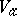be the germ at a pointof a real-analytic subset of(cf. Analytic set). This defines the germ atof a complex-analytic subset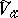of the spacewith the following equivalent properties: 1)is the intersection of all germs of complex-analytic sets containing; 2) if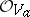is the analytic algebra of the germ, then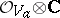is the analytic algebra of the germ. The germis said to be the complexification of the germ, andis said to be the real part of the germ. Analogously, for any coherent real-analytic countably-infinite spaceit is possible to construct the complexification, which is a complex-analytic space.will then have a fundamental system of neighbourhoods in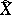which are Stein spaces (cf. Stein space).

The theory of coherent real-analytic spaces is similar to the theory of complex Stein spaces. Global sections of any coherent analytic sheaf of moduleson a coherent real-analytic countably-infinite spacegenerate modules of germs of its sections at any point of, and all groups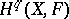vanish if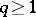.

For any finite-dimensional coherent real-analytic countably-infinite space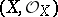there exists a morphism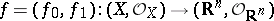such that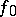is a proper one-to-one mapping ofinto a coherent subspace in, whileis an imbedding at the smooth points of. In particular, any (Hausdorff and countably-infinite) real-analytic manifold is isomorphic to a real-analytic submanifold in. For a reduced coherent real-analytic spacethe set of classes of isomorphic real-analytic principal fibre bundles with a real structure Lie group, admitting complexification, and baseis in one-to-one correspondence with the set of classes of isomorphic topological principal fibre bundles with the same structure group.

How to Cite This Entry:
Real-analytic space. Encyclopedia of Mathematics. URL: http://encyclopediaofmath.org/index.php?title=Real-analytic_space&oldid=14527
This article was adapted from an original article by D.A. Ponomarev (originator), which appeared in Encyclopedia of Mathematics - ISBN 1402006098. See original article